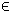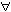## 3MILP Problem

The general formulation in TOMLAB for a mixed-integer linear programming problem is:

 min x
f(x) = cT x

s/t
 xL ≤ x ≤ xU, bL ≤ A x ≤ bU,  xjNjI
(3)
where c, x, xL, xURn, ARm1 × n, and bL,bURm1. The variables xI, the index subset of 1,...,n are restricted to be integers. Equality constraints are defined by setting the lower bound equal to the upper bound, i.e. for constraint i: bL(i) = bU(i).

Mixed-integer linear problems are defined in the same manner as linear problems. However, the user can give a wider range of inputs to the assign routine and solvers. See 'help mipAssign' for more information. In TOMLAB integers can be identified by a 0-1 vector.

The following example illustrates how to solve a MILP problem using the TOMLAB format.

File: tomlab/quickguide/mipQG.m

Open the file for viewing, and execute mipQG in Matlab.
```% mipQG is a small example problem for defining and solving
% mixed-integer linear programming problems using the TOMLAB format.

Name='Weingartner 1 - 2/28 0-1 knapsack';
% Problem formulated as a minimum problem
A = [ 45      0     85     150     65     95     30      0    170  0 ...
40     25     20       0      0     25      0      0     25  0 ...
165     0     85       0      0      0      0    100  ; ...
30     20    125       5     80     25     35     73     12  15 ...
15     40      5      10     10     12     10      9      0  20 ...
60     40     50      36     49     40     19    150];
b_U = [600;600];  % 2 knapsack capacities
c   = [1898  440  22507   270  14148  3100  4650  30800   615  4975 ...
1160 4225    510 11880    479   440   490    330   110   560 ...
24355 2885  11748  4550    750  3720  1950  10500]'; % 28 weights

% Make problem on standard form for mipSolve
[m,n]   = size(A);
c       = -c;           % Change sign to make a minimum problem
x_L     = zeros(n,1);
x_U     = ones(n,1);
x_0     = zeros(n,1);

fprintf('Knapsack problem. Variables %d. Knapsacks %d\n',n,m);

% All original variables should be integer
IntVars = n;   % Could also be set as: IntVars=1:n; or IntVars=ones(n,1);
x_min   = x_L; x_max  = x_U; f_Low  = -1E7; % f_Low <= f_optimal must hold
b_L     = -inf*ones(2,1);
f_opt   = -141278;

nProblem  = []; % Problem number not used
fIP       = []; % Do not use any prior knowledge
xIP       = []; % Do not use any prior knowledge
setupFile = []; % Just define the Prob structure, not any permanent setup file
x_opt     = []; % The optimal integer solution is not known
VarWeight = []; % No variable priorities, largest fractional part will be used
KNAPSACK  = 1;  % Run with the knapsack heuristic

% Assign routine for defining a MIP problem.
Prob      = mipAssign(c, A, b_L, b_U, x_L, x_U, x_0, Name, setupFile, ...
nProblem, IntVars, VarWeight, KNAPSACK, fIP, xIP, ...
f_Low, x_min, x_max, f_opt, x_opt);

Prob.optParam.IterPrint = 0; % Set to 1 to see iterations.
Prob.Solver.Alg = 2;   % Depth First, then Breadth search

% Calling driver routine tomRun to run the solver.
% The 1 sets the print level after optimization.

Result = tomRun('mipSolve', Prob, 1);
%Result = tomRun('cplex', Prob, 1);
%Result = tomRun('xpress-mp', Prob, 1);
%Result = tomRun('miqpBB', Prob, 1);
%Result = tomRun('minlpBB', Prob, 1);```